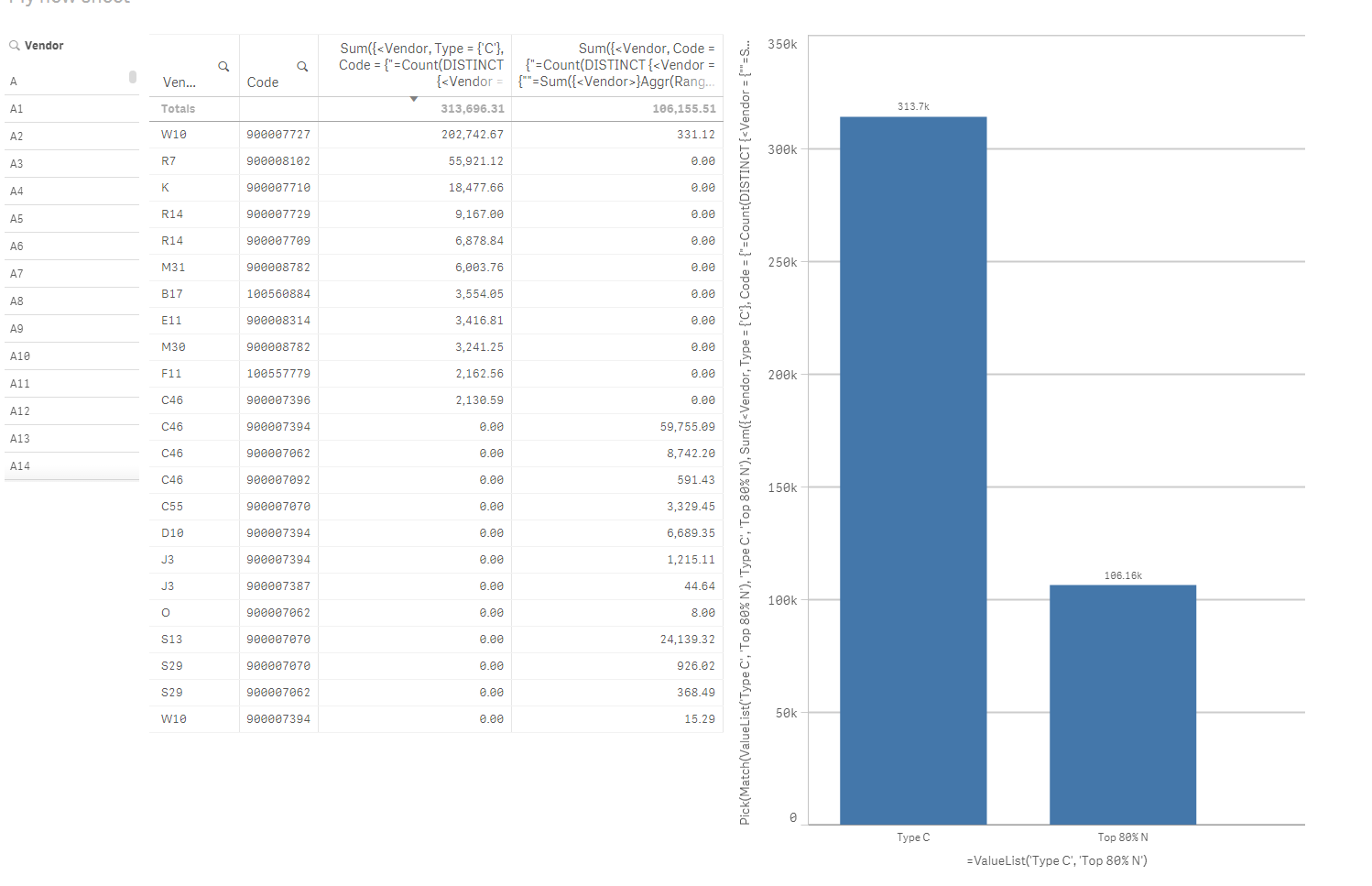# Qlik Sense App Development

Announcements
Save the Date: QlikWorld Online, June 24-25, 2020. Free global virtual event for data integration and data analytic gurus. Register Today
HighlightedMVP

## Re: Create a calculated Pareto dimension involving Select

What rows constitutes of the 11,407.56?

Highlighted
Contributor III

## Re: Create a calculated Pareto dimension involving Select

Sales should be 11,407.56 under Type C. That is the final result.

HighlightedMVP

## Re: Create a calculated Pareto dimension involving Select

I get that, but which Vendors are you including in your total sales? Currently, C2, C3, D, & F were getting included... Are those not right?

Highlighted
Contributor III

## Re: Create a calculated Pareto dimension involving Select

Apparently not. So to make it clear, here's how I'd do it in excel:

1.Create a Pareto on the Overall Vendors. Select the fields which are of Type='N' and Type1='M' which fall under Bottom 20% Pareto.

2.Note the Codes of all these fields which are present after the above filters are applied.

3. Cross check only these codes against the Codes field in the entire data set.

4. See how much Sales of these fields fall under the Type 'C' or Top 80% N.

Highlighted
Contributor III

## Re: Create a calculated Pareto dimension involving Select

It's okay if you don't get the matching result too. Just giving me the expression would be enough. Thanks!

Highlighted
Contributor III

## Re: Create a calculated Pareto dimension involving Select

Hello. Here's the entire dataset. You can also find calculations done in the sheet. So to reiterate what I want.

1.Perform a Pareto analysis on the whole dataset Vendors.

2.Select the Vendors in the Bottom 20%. Within the transactions of these Bottom 20% Vendors, note the Codes which have Type='N' and Type1='M'.

3.Now cross check these noted codes from above against the entire dataset.

4.See how much of their values fall under the Type 'C' or Type 'Top 80% Pareto Vendors within Type N'.

The result for this dataset should be: Type 'C':\$310,142.26 and Top 80% N:\$ 106,552.52MVP

## Re: Create a calculated Pareto dimension involving Select

Here you areDimension

=ValueList('Type C', 'Top 80% N')

Expression

Pick(Match(ValueList('Type C', 'Top 80% N'), 'Type C', 'Top 80% N'),

Sum({<Vendor, Type = {'C'},

Code = {"=Count(DISTINCT {<Vendor = {""=Sum({<Vendor>}Aggr(Rangesum(Above(Sum({<Vendor>} Sales)/Sum({<Vendor>} TOTAL Sales), 0, RowNo())), (Vendor,(=Sum({<Vendor>} Sales),Desc)))) >= 0.8""}, Type = {'N'}, Type1 = {'M'}>}Code) >= 1"}>} Sales),

Sum({<Vendor,

Code = {"=Count(DISTINCT {<Vendor = {""=Sum({<Vendor>}Aggr(Rangesum(Above(Sum({<Vendor>} Sales)/Sum({<Vendor>} TOTAL Sales), 0, RowNo())), (Vendor,(=Sum({<Vendor>} Sales),Desc)))) > 0.8""}, Type = {'N'}, Type1 = {'M'}>}Code) >= 1"},

Vendor = {"=Sum({<Vendor>}Aggr(Rangesum(Above(Sum({<Vendor>} Sales)/Sum({<Vendor>} TOTAL Sales), 0, RowNo())), (Vendor,(=Sum({<Vendor>} Sales),Desc)))) <= 0.8"},

Type = {'N'}>} Sales))

Also, please find attached the application with this email.

Highlighted
Contributor III

## Re: Create a calculated Pareto dimension involving Select

Awesome! This is amazing stuff! I can't thank you enough!